Home | | Maths 8th Std | Exercise 1.6 (Exponents and Powers)

# Exercise 1.6 (Exponents and Powers)

8th Maths : Chapter 1 : Numbers : Exponents and Powers : Exercise 1.6 : Text Book Back Exercises Questions with Answers, Solution

Exercise 1.6

1. Fill in the blanks:

(i) (−1)even integer is _____________. [Answer:1]

(ii) For a ≠ 0, a0 is ______________. [Answer:1]

(iii) 4-3 × 5-3 __________. [Answer:20−3]

(v) (-1/3)-5 = ____________. [Answer: −243]

2. Say True or False:

(i) If 8x = 1/64, the value of x is − 2. [Answer: True]

(ii) The simplified form of (256)−1/4 × 42 is ¼. [Answer: False]

(iii) Using the power rule, (37)-2 = 35. [Answer: False]

(iv) The standard form of 2 × 10–4 is 0.0002. [Answer: True]

(v) The scientific form of 123.456 is 1.23456×102. [Answer: False]

3. Evaluate: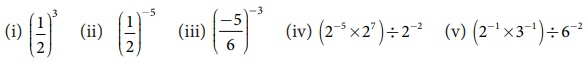(i) (1/2)3

Solution:

(1/2)3 = 13/23 = 1 / [2 × 2 × 2] = 1 / 8

(ii) (1/2)−5.

Solution:

(1/2)−5 = 1−5 / 2−5 = 1 / 2−5 = 25 = 2 × 2 × 2 × 2 × 2 = 32

(iii) (−5/6)−3

Solution:(−5/6)−3 = (−5)−3 / 6−3 = 63 / (−5)−3 = ( (6 × 6 × 6) / (−5 × −5 × −5) ) = − 216/125

(iv) (2−5 × 27) ÷ 2−2

Solution:

(2−5 × 27) ÷ 2−2 = (2−5+7) ÷ 2−2

= 22 ÷ 2−2

= 22+2

= 24 = 16

(v) (2−1 × 3−1) ÷ 6−2

Solution:

(2−1 × 3−1) ÷ 6−2 = (2 × 3)−1 ÷ 6−2

= (6)−1 ÷ 6−2

= (6)−1 – (−2) = 61 = 6

4. Evaluate:Solution:(2/5)4 × (2/5)2 = (2/5)4+2  =  (2/5)6

(ii) (4/5)−2 ÷ (4/5)−3 = (4/5)−2 × (4/5)3  = (4/5)−2+3  = (4/5)−2+3  = (4/5)1 = 4/5

(iii) 27 × (1/2)−3 = 27 × 23 = 27+3 = 210 = 1024

5. Evaluate: (i) (50 +6-1) × 32 (ii) (2-1 + 3-1 ) ÷ 6-1 (iii) ( 3-1 + 4-2 + 5-3)0

Solution: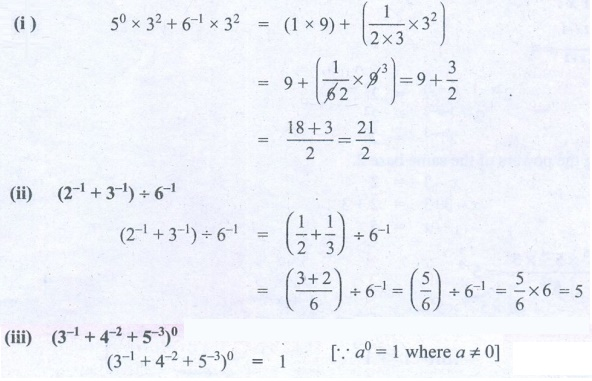(i) (50 +6−1) × 32

50 × 32 + 6−1 × 32 = (1 × 9) + ( {1 / [2×3]} × 32)

= 9 + ( 1/6 × 9 ) = 9 + 3/2

= [18 + 3] / 2 = 21/2

(ii) (2−1 + 3−1) ÷ 6−1

(2−1 + 3−1) ÷ 6−1 = (1/2 + 1/3) ÷ 6−1

= ( [3 + 2] / 6) ÷ 6−1  = (5/6) ÷ 6−1 = 5/6 × 6 = 5

(iii) (3−1 + 4−2 + 5−3)0

(3−1 + 4−2 + 5−3)0 = 1

[  a0 = 1 where a ≠ 0]

6. Simplify: (i) (32)3 × (2×35)–2 × (18)2Solution: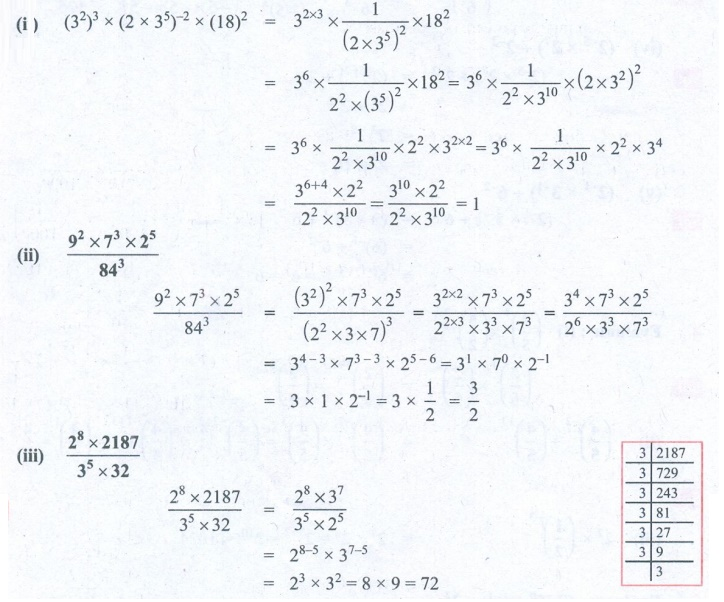(i) (32)3 × (2 × 35)−2 × (18)2

(32)3 × (2 × 35)−2 × (18)2 = 32x3 × [1 / (2 × 35)2 ] × 182

= 36 × [1 / 22×(35)2 ] × 182 = 36  × [ 1 / 22×310 ] × (2×32)2

= 36  × [ 1 / 22×310 ] × 22 × 32×2 =  36 × [ 1 / 22×310 ] × 22 × 34

= [36+4  × 22 ] / [22 × 310 ] = [310 × 22 ] / [ 22 × 310 ] = 1

(ii) [ 92 × 73 × 25 ] / 843

[ 92 × 73 × 25 ] / 843 = [ (32)2 × 73 × 25 ] / [ (22 × 3 × 7)3 ] = [ 32x2 × 73 × 25 ] / [ 22x3x 33 × 73 ] = [ 34 × 73 × 25 ] / [ 26 × 33 × 73 ]

= 34−3 × 73−3 × 25−6 = 31 × 70 × 2−1

= 3 × 1 × 2−1 = 3 × (1/2) = 3/2

(iii) [ 28 × 2187] / [35 × 32]

Solution:[28 × 2187] / [35 × 32] = [28 × 37 ] / [ 35 × 25]

= 28−5 × 37−5

= 23 × 32 = 8 × 9 = 72

7. Solve for x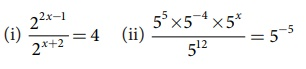(i) 22x−1 / 2x+2 = 4

Solution:

22x−1 –(x+2) = 22

22x−1 x−2) = 22

2x−3 = 22

Equating the powers of the same base 2.

x−3 = 2

x – 3 + 3 = 2 + 3

x = 5

(ii) [ 55 × 5−4 × 5x ] / 512 = 5−5

[ 55 × 5−4 × 5x ] / 512 = 5−5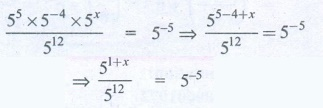⇒ [ 55 −4 + x ] / 512 = 5−5

51+ x / 512 = 5−5

51+ x − 12 = 5−5

5 x −11 = 5−5

Equating the powers of same base 5.

x − 11 = −5

x —11 + 11 = −5 + 11

x = 6

8. Expand using exponents: (i) 6054.321 (ii) 897.14

(i) 6054.321 = (6 × 1000) + (0 × 100) + (5 × 10) + (4 × 100) + 3/10 + 2/100 + 1/1000

= (6 × 103) + (5 × l01) + (4 × 100) + ( 3 × 1/10) + ( 2 × 1/100) + (1 × 1/1000)

= (6 × 103) + (5 × l01) + (4 × 100) + ( 3 × 10−1) + ( 2 × 10−2) + (1 × 10−3)

(ii) 897.14 = (8 × 100) + (9 × 10) + (7 × 100) + l/10 + 4/100

=  (8 × 102) + (9 × 101) + (7 × 100) + (l × 1/10) + (4 × 1/100)

=  (8 × 102) + (9 × 101) + (7 × 100) + (l × 10−1) + (4 × 10−2)

9. Find the number in standard form for the following expansions:

(i) 8×104 +7×103 +6×102 +5×101 +2×1+ 4×10-2 +7×10-4

(ii) 5×103 + 5×101 + 5×10–1 + 5 ×10–3

(iii) The radius of a hydrogen atom is 2.5 × 10–11 m

Solution:

(i) 8 × 104 + 7 × 103 + 6 × 102  + 5 × 101 + 2 × 1 + 4 × 10−2 + 7 × 10−4

(8 × 104) + (7 × 103) + (6 × 102)  + (5 × 101) + (2 × 1) + (4 × 10−2) + (7 × 10−4)

= (8 × 10000) + (7 × 1000) + (6 × 100) + (5 × 10) + (2 × 1) + (4 × 1/100) + (7 × 1/10000)

= 80000 + 7000 + 600 + 50 + 2 + (4/100) + (7/10000)

= 87652.0407

(ii) 5 × 103 + 5 × 101 + 5 × 10−1 + 5 × 10−3

Solution:

5 × 103 + 5 × 101 + 5 × 10−1 + 5 × 10−3

= 5 × 1000 + 5 × 10 + 5 × 1/10 + 5 × 1/1000

= 5000 + 50 + 5/10 + 5/1000 = 5050.505

(iii) The radius of a hydrogen atom is 2.5 × 10−11 m.

Solution:

Radius of a hydrogen atom = 2.5 × 10−11 m

= 2.5 × 1/1011 m = 2.5/1011m = 0.000000000025 m

10. Write the following numbers in scientific notation:

(i) 467800000000 (ii) 0.000001972 (iii) 1642.398

(iv) Earth’s volume is about 1,083,000,000,000 cubic kilometres

(v) If you fill a bucket with dirt, the portion of the whole Earth that is in the bucket will be 0.0000000000000000000000016 kg

Solution:Objective Type Questions

11. By what number should (−4)1 be multiplied so that the product becomes 10-1 ?

(A) 2/3

(B) −2/5

(C) 5/2

(D) −5/2

Solution:(−4)−1 = (−1/4)1 = −1/4

10−1 = (1/10)1 = 1/10

(−1/4) × x = 1/10

x = −4 / 10 = −2 / 5

12. (−2)−3 × (−2 )−2 = ____________.

(A) −1/32

(B) 1/32

(C) 32

(D) –32

13. Which is not correct?Solution:

(−2) – 3x( − 2) – 2 = (−2) – 3 – 2 = (−2) −5 (−1/2)5 = −1/32

14. If 10x/10-3 =109 ,then x is ____________.

(A) 4

(B) 5

(C) 6

(D) 7

15. 0.0000000002020 in scientific form is ____________.

(A) 2.02×109

(B) 2.02×109

(C) 2.02×108

(D) 2.02×1010

Solution:Exercise 1.6

1. (i) 1 (ii) 1 (iii) 20−3 (iv) −1/128 (v) –243

2. (i) True  (ii) False  (iii) False  (iv) True  (v) False

3. (i) 1/8  (ii) 32  (iii) – 216/125  (iv) 16  (v) 6

4. (i) 64/15625  (ii) 4/5  (iii) 1024

5. (i) 21/2 (ii) 5 (iii) 1

6. (i) 1  (ii) 3/2  (iii) 72

7. (i) x = 5  (ii) x = 6

8. (i) 6 × 103 + 5 × 101 + 4 × 100 + 3 × 10‒1 + 2 × 10‒2 + 1 × 10-3

(ii) 8 × 102 + 9 × 101 + 7 × 100 + 1 × 10‒1 + 4 × 10‒2

9. (i) 87652.0407 (ii) 5050.505 (iii) 0.000000000025

10. (i) 4.678 × 1011 (ii) 1.972 × 10‒6 (iii) 1.642398 ×103 (iv) 1.083 ×1012 cu. km (v) 1.6 ×10‒24

11. (B)  -2/5

12. (A)  -1/32

13. (D) – (1/4)2 = 16-1

14. (C) 6

15. (D) 2.02×10-10

Tags : Questions with Answers, Solution | Numbers | Chapter 1 | 8th Maths , 8th Maths : Chapter 1 : Numbers
Study Material, Lecturing Notes, Assignment, Reference, Wiki description explanation, brief detail
8th Maths : Chapter 1 : Numbers : Exercise 1.6 (Exponents and Powers) | Questions with Answers, Solution | Numbers | Chapter 1 | 8th Maths